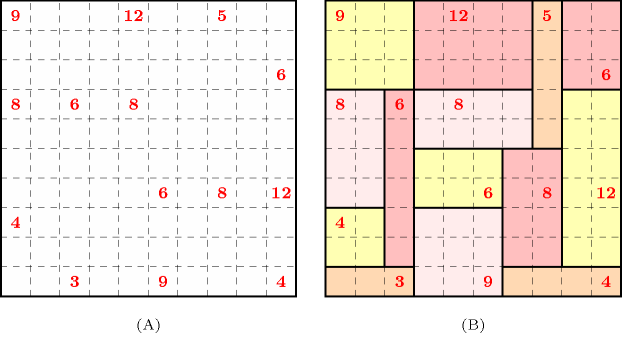### 3.7.223. Shikaku

Denotes that a constraint can be used for solving the Shikaku puzzle. Given a rectangular grid, where exactly $n$ cells contain an integer value, the problem is to tile that grid by $n$ rectangles in such a way that the surface of each rectangle is equal to the single integer it contains.

##### Figure 3.7.59. (A) An example of a Shikaku puzzle and (B) its corresponding unique solutionParts (A) and (B) of Figure 3.7.59 respectively show a small instance of such a puzzle and its corresponding unique solution taken from the Nikoli website https://member.nikoli.com/index.html.